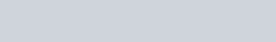In this tutorial you are shown the formulae that are used to calculate the mean, E(X) and the variance Var(X) for a continuous random variable by comparing the results for a discrete random variable. There is a brief reminder of what a discrete random variable is at the start.

Mean E(X) and Variance Var(X) for Continuous Random Variables : ExamSolutions - youtube Video

Example

In this next example you are asked to calculate the mean and variance of a continuous random variable. Again it would be a good one to try first and then check your work against the worked solution. I would strongly suggest looking at the video anyway as there is a simple way of finding the mean for this example which you should be aware of.

A continuous random variable X has a probability density function f(x) given byFind the mean E(X) and the variance Var(X).

Example | Mean E(X) and Variance Var(X) for a Continuous Random Variable - youtube Video